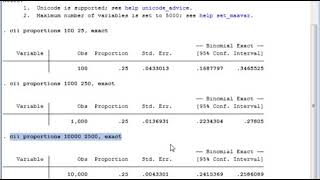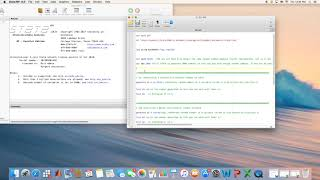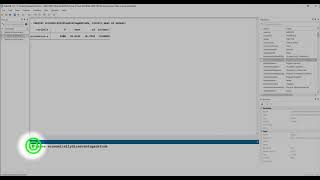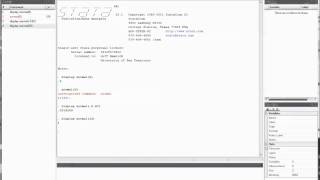More Stata Tips & Tutorials: Select Page ... Getting Started with Stata Data Analysis with Stata Descriptive Statistics Creating and Transforming Variables Making Comparisons Graphing Relationships and Describing Patterns Random Assignment and Sampling Making Controlled Comparisons Foundations of Statistical Inference Hypothesis Tests with One and Two Samples Chi-Square and Analysis of Variance Correlation and Bivariate Regression Multiple Regression Analyzing Regression Residuals Logistic Regression Doing Your Own Political Analysis Advanced Political Analysis with Stata
Watch and Learn
Watch and LearnStata confidence interval of proportions272analytics (5:11)Generating Random Variables Using Uniform, Normal DistributionsNEmara (7:24)Using Stata Constructing a Confidence IntervalJason Burns (6:44)Computing Normal Probabilities with StataJeff Hamrick (3:57)Stata confidence interval of proportions272analytics (5:11)Generating Random Variables Using Uniform, Normal DistributionsNEmara (7:24)